You will get a review of basic arithmetic calculations in this section including:

## Basic Terms and Terminology Relating to Arithmetic Operations with Rational Numbers

• Associative and commutative processes: Those mathematical processes and calculations that can be accurately done regardless of the order of the numbers.
• Addition: An associative and commutative process that combines numbers together. Addition is the opposite of subtraction and it has some similarities with multiplication.
• Subtraction: The opposite of addition and the basic arithmetic calculation that takes one number away from another; subtraction is not commutative and it is not associative and it shares some conceptual similarities with division.
• Difference: The answer to a subtraction calculation. Minuend �' Subtrahend = Difference
• Minuend: The number in the subtraction calculation that is decreased and "taken away from "by the subtrahend. The minuend is the top number in a subtraction calculation. Minuend �' Subtrahend = Difference
• Subtrahend: The number in the subtraction calculation that is "taken away from" the minuend. The subtrahend is the bottom number in a subtraction calculation. Minuend �' Subtrahend = Difference
• Multiplication: An associative and commutative process that combines numbers together with repeated addition which is simplified with the use of the multiplication tables. Multiplication is the opposite of division and it has some similarities with addition.
• Product: The answer to a multiplication calculation.
• Division: A mathematical and arithmetic process that divides a number into equal parts. Division is not commutative and it is not associative and it shares some conceptual similarities with subtraction.
• Dividend: The number in a division calculation that is divided up into equal parts. Dividend �- Divisor = Quotient
• Divisor: The number in a division calculation that is divided into the dividend. Dividend �- Divisor = Quotient
• Quotient: The answer to a division calculation; Dividend �- Divisor = Quotient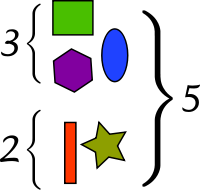Possibly the most fundamental interpretation of addition lies in combining sets.

Addition is the least complex and simplest of the four basic arithmetic calculations. Addition is also the basis of multiplication and the opposite of subtraction. Although most, if not all of us, are able to add relatively simple whole numbers, it is often difficult for some of us to add more complex numbers like decimals, fractions and mixed numbers.

Before we address complex addition, it may be helpful to you to more fully understand some of the underlying mathematical concepts that underlay addition.Plus Sign

Addition is an associative and commutative process. The associative process indicates that addition will consistently render the same answer regardless of how the order of the numbers are added together and the commutativity of addition indicates that order of the numbers do not matter in terms of the sum. A sum is the answer that you get after adding numbers together.

As young children develop, they use concrete objects to learn the concepts underlying basic arithmetic operations, so we will include some concrete objects so that you may be able to more fully understand these arithmetic concepts that are presented in this section.

Addition in a concrete manner is the joining of objects and sets of objects. As shown in the picture above, a set of 2 and a set of 3 will give you a total of 5 in both sets when they are combined, or added together.

Other concrete operations relating to addition can include things like adding money together and adding the number of apples on the table, as shown below: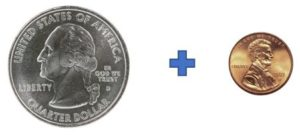25 cents + 1 cent + 25 cents = 51 cents1 apple + 2 apples + 2 apples = 5 apples

Addition calculations and operations using abstract numbers, rather than concrete objects is done as shown below:

6 + 2 = 8

12 + 34 + 22 should be done as below: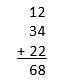Note that these numbers in a different order, as shown above, will render the same sum of 68.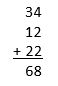127 + 32 + 220 should be done as below: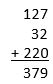Notice again, that these same numbers in a different order will yield the same sum of 695.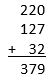As you can see in the examples above, the ones, tens and hundredths of each number is lined up in these calculations.

The ones place is the last place of a multiple digit number, the tens place is the second to last place of a multiple digit number and the hundreds place is the third to the last place of a multiple digit number. So, in the number 379, 9 is in the ones place, 7 is in the tens place and 3 is in the hundreds place.

For example, the ones place for each of the numbers above in this addition calculation, from the top number to the bottom number are 0, 7 and 2 respectively; the tens place in each of the numbers above in this addition calculation, from the top number to the bottom number are 2, 2 and 3; and the hundreds place each of the numbers above in this addition calculation, from the top number to the bottom number are 2 and 1; the last digit 32 does not have a hundreds place.

Some addition calculations require that we "carry over" a number to the next place. The example below shows an addition calculation that does NOT require that we "carry over" because each of the columns, that is the ones, the tens and the hundreds place do NOT add up to 10 or more.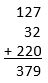The addition calculation below, however, requires that we "carry over" a number to the next place because the sum of the tens place is more than 10. See the 1 that was carried over to the hundreds place so that the hundreds place numbers are now 1 + 1 + 2 for a total of 4.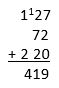Here is another addition calculation with "carry overs":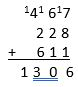Remember to use your calculator during the TEAS. Careless errors can be made with even the simplest of addition calculations.

## Subtraction

Subtraction is the opposite of addition; in fact, you can check your answer for subtraction which is called the difference, by adding the difference to one of the numbers you used in the subtraction calculation.

Subtraction, in contrast to addition, is not commutative and it is not associative. The order of the numbers for subtraction calculations DOES matter; the order of the numbers for addition, as shown above, do not matter.

Again, in terms of concrete objects, subtraction concepts can be understood by taking or removing 2 oranges from the table where there were 5 oranges, as shown below.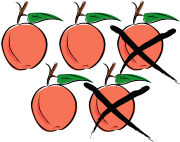5 - 2 = 3 oranges

As you can see, there are 3 oranges left when you remove 2 oranges off the table.

Similarly, 25 cents - 1 cent = 24 cents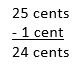The answer in subtraction is referred to as the difference. The number on the top of a subtraction problem is called the minuend and the number on the bottom is referred to as the subtrahend. The minuend is the number that you are subtracting FROM and the minuend is the number that you are subtracting from the minuend. For example, in the subtraction calculation above, the difference is 24 cents, the minuend is 25 cents and the subtrahend is 1 cent.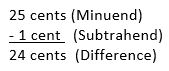When the minuend is equal to or greater than the subtrahend, the difference will be a zero or a positive number, respectively. However, when the minuend is less than the subtrahend, the answer to the subtraction calculation will be a negative number that is less than zero.

The example above shows subtraction where the minuend is greater than the subtrahend; the difference, therefore, is a positive number.

The example below shows subtraction when the minuend is equal to the subtrahend. As you can see the difference is zero (0) when the minuend is equal to the subtrahend.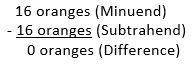The example below shows subtraction when the minuend is less than the subtrahend. As you can see, the answer to the subtraction calculation is a negative number that is less than zero.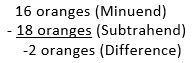### Checking Subtraction Calculations

You can double check your subtraction calculations by adding the difference to the number that you subtracted, the subtrahend, and then compare this number to the top number in the subtraction that was subtracted from, the minuend, as shown below.

For example, 3 oranges + 2 oranges = 5 oranges and 24 cents + 1 cent = 25 cents. See the examples below: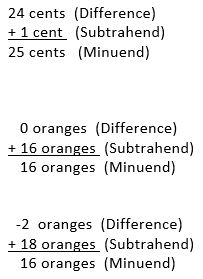Here are some more simple subtraction calculations and rechecking these calculations with the inverse operation of addition: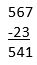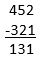More complex subtraction calculations require that we "borrow" from the next place. "Borrowing" is necessary when the number that you are subtracting is MORE than the number that you are subtracting from.

Here are some examples: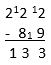By adding the difference (133) to the subtrahend (89), the answer is 222, as shown above as the minuend. Checking the answer with addition entails adding 133 to 89. The answer for this addition is 222, therefore, the subtraction above is accurate because checking it with the inverse operation of addition gives you the minuend of 222.

In the above example, 9 could not have been subtracted from 2, therefore, you would have to "borrow" 10 more from the tens column and then add it to the making the 2 a 12 and making the 8 in the tens column a 9, The same "borrowing" and "paying back" is then done again for the tens place.

Here are some more examples of more complex subtraction calculations: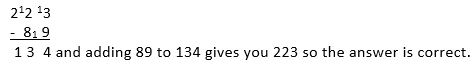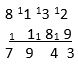Remember to use your calculator during the TEAS. Careless errors can be made with even the simplest of subtraction calculations.

## Multiplication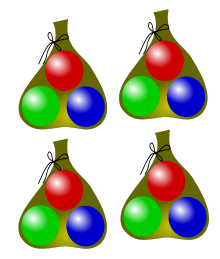Four bags with three marbles per bag gives twelve marbles (4 �- 3 = 12).

Multiplication is somewhat similar to addition. Both processes are associative and commutative processes. Additionally, multiplication is conceptually the repeated addition of the same number. For example, when you look at the marbles in the picture above, you could do one of three things, as follows.

1. Count all the individual marbles and get to a total of 12
2. Add 3 + 3 + 3 + 3 = 12 or
3. Multiply 4 sets with 3 in each set or bag. 4 x 3 = 12

Multiplication is the opposite of division and the inverse operation for division.

Multiplication problems and calculations can be done with the memorization of the multiplication tables and they can also be done using the x function on the calculator. The answer to a multiplication calculation is called the product.

Here are some simple multiplication calculations:

• 3 x 2 = 6
• 2 x 3 = 6
• 9 x 5 = 45
• 8 x 6 = 48
• 11 x 4 = 44

Below are some examples of multiplication that are more complex than the ones above.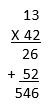Step 1:

As you can see in the above example, both 13 and 42 are lined up according to the tens and ones places.

Step 2:

After that is set up, you would multiply the 2 in the ones place times the 3 in the ones place and then you would multiply the same 2 in the ones place times the 1 in the tens place.

Step 3:

Next, you have to multiply the 4 in the tens place by both the 3 in the ones place and the 1 in the tens place but when you perform this multiplication, you have to begin this second line into the tens place and NOT the ones place because you are now multiplying by the 4 in the tens place and NOT in the ones place.

Step 4 and the Final Step:

Add the two numbers together and you will get the answer of 546.

Here are some other examples of multiplication using the 4 steps as discussed above.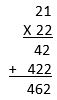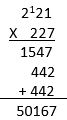### Multiplying by Ten and Multiples of Ten

Although you can use a calculator during your TEAS examination, it is still helpful to understand the basic, and very simple, way to multiply and divide by ten and multiples of ten.

The multiples of ten are:

• 10
• 100
• 1,000
• 10,000
• 100,000
• 1,000,000 etc.

When multiplying by 10, you would simply place one zero (0) after the number. Here are some examples:

• 4 x 10 = 40
• 45 x 10 = 450
• 579 x 10 = 5,790
• 5634 x 10 = 56,340

When multiplying by 100, you would simply place two zeros (0) after the number. Here are some examples:

• 4 x 100 = 400
• 45 x 100 = 4,500
• 579 x 100 = 57,900
• 5634 x 100 = 563,400

When multiplying by 1,000, you would simply place three zeros (0) after the number. Here are some examples:

• 4 x 1000 = 4,000
• 45 x 1000 = 45,000
• 579 x 1000 = 579,000
• 5634 x 1000 = 5,634,000

When multiplying by 10,000, you would simply place four zeros (0) after the number. Here are some examples:

• 4 x 1000 = 40,000
• 45 x 1000 = 450,000
• 579 x 1000 = 5,790,000
• 5634 x 1000 = 56,340,000

By now, you may have seen a pattern. Did you notice that when you multiplied by 10, you added one zero and that there is one zero in the number 10? Did you notice that when you multiplied by 100, you added two zeros and that there are two zeros in the number 100? We hope you did.

Based on this observation, the easiest way to multiply by 10 and multiples of 10 i8s to count the number of zeros in the multiples of ten and add this number of zeros to the number that you are multiplying by 10 or the multiple of ten.

So, 23 x 1,000,000,000,000 is:

23,000,000,000,000 with 9 zeros

## Division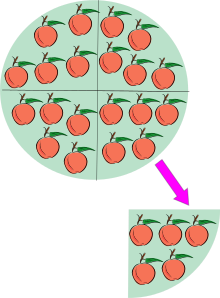20 �- 5 = 4 because 20 apples contain five apples four times. That is an example of division as a quotient rather than as partition. One can also say that 20 �- 5 = 4 because when 20 apples are divided into 5 equal sets of apples, then there are 4 in each such set. That is division as partition rather than as a quotient.

In some respects, division is similar to subtraction, in the same manner that multiplication is similar to addition. Both subtraction and division are not commutative and not associative; and division is repeated subtraction in somewhat the same manner as multiplication is repeated division.

The answer to a division calculation is called the quotient; the number that is being divided up is called the dividend and the number that is divided into the dividend is called the divisor.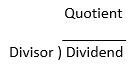Conceptually, division is taking a group of items such as the apples above, dividing it or breaking it up into equal sets, and then determining how many equal sets result.

For example, in the picture above, the 20 apples that are divided into equal sets of 5 apples in each set, result in 4 equal sets of five.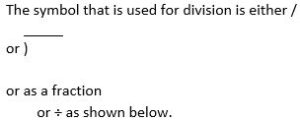For example: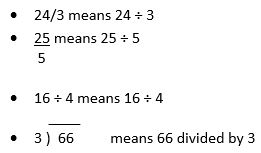Some division calculations, like the ones above, are evenly divided without anything left over. But, there are many division calculations that do result in a remainder, as shown below.

For example, 16 �- 4 = 4 is divided evenly without anything left over, however, 18 �- 4 = 4 and 2 left over which cannot be put into another equal set of 4. The answer to this division problem with the remainder of 2 can be expressed in several ways.

• 18 �- 4 = 4 2/4 or 4 and 2 quarters which is 4 �1/2 when the 2/4 is reduced
• Or 18 �- 4 = 4.5

Similar to subtraction, answers to division problems can be checked by multiplying the answer, or quotient, by the divisor which should give you the dividend as shown in the examples below.

18 �- 4 = 4.5

Checking with Multiplication: 4.5 x 4 = 18

24/3 = 8

Checking with Multiplication: 8 x 3 = 24

25 = 5
5

Checking with Multiplication: 5 x 5 = 25

16 �- 4 = 4

Checking with Multiplication: 4 x 4 = 16

____
3 ) 66 = 22

Checking with Multiplication: 22 x 43 = 66

### Long Division

More complicated division calculations are called long division. Here are some examples of long division are shown below.

321/ 22 = 14.59 and this quotient can be verified as correct when you multiply the quotient, or answer, by the divisor of 22 (14.59 x 22 = 321)

687 = 45.8
15 and this quotient can be verified as correct when you multiply the quotient, or answer, by the divisor of 15 (45.8 x 15 = 687)

89 �- 16 = 5.56 and this quotient can be verified as correct when you multiply the quotient, or answer, by the divisor of 16 (5.56 x 16 = 89 when rounded off)

666/22 = 30.27 and this quotient can be verified as correct when you multiply the quotient, or answer, by the divisor of 22 (30.27 x 22 = 666)

257 = 4.94
52 and this quotient can be verified as correct when you multiply the quotient, or answer, by the divisor of 52 (4.94 x 52 = 257)

123 �- 21 = 5.86 rounded off and this quotient can be verified as correct when you multiply the quotient, or answer, by the divisor of 21 (5.86 x 21 = 123)

908/ 134= 6.78 rounded off and this quotient can be verified as correct when you multiply the quotient, or answer, by the divisor of 134 (6.78 x 134 = 908 rounded off)

32 = 6.4
5 and this quotient can be verified as correct when you multiply the quotient, or answer, by the divisor of 5 (6.4 x 5 = 32)

889 �- 124 = 7.17 and this quotient can be verified as correct when you multiply the quotient, or answer, by the divisor of 124 (7.17 x 124 = 889)

### Dividing by Ten and Multiples of Ten

Dividing by 10 and multiples of ten is the opposite of multiplying by ten and multiples of ten. When you divide by ten and multiples of ten, you cross off and eliminate the same number of zeros that are in the number you are dividing by OR you move the decimal point to the left one space for each zero in the multiple of ten that you are dividing by.

Here are some examples:

• 20 �- 10 = 20 or 2
• 360 �- 10 = 360 or 36
• 235,000 �- 10 = 235000 or 23,500
• 200 �- 100 = 200 or 2
• 360,000 �- 100 = 360000 or 3,600
• 2,350,000 �- 100 = 2350000 or 23,500
• 20,000 �- 1000 = 20000 or 20
• 360,000,000 �- 1000 = 360000000 or 360,000
• 2,350,000 �- 1000 = 2350000 or 2,350

All of the above examples have enough zeros to enable you to simply cross off or eliminate the number of zeros needed to do these division calculations, but there are many cases when dividing by 10 and multiples of 10 does not occur like this.

For example, the division examples with multiples of ten without sufficient zeros includes calculations such as those shown below:

• 560 �- 100
• 62 �- 10
• 62 �- 100
• 234 �- 1,000
• 22 �- 10,000

In these cases, the calculations are done according to the same mathematical principles that were discussed above. For example, when you divide by 10, you cross off one zero and when you divide by 100 you cross off two zeros.

When numbers have no zeros and when numbers have less than the necessary zeros to cross off, you will move the decimal place of the number:

• 1 place to the left and cross off any zeros while leaving all non zero numbers in place with the decimal point when you are dividing by 10.
• 2 places to the left and cross off any zeros while leaving all non zero numbers in place with the decimal point when you are dividing by 100.
• 3 places to the left and cross off any zeros while leaving all non zero numbers in place with the decimal point when you are dividing by 1,000.
• 4 places to the left and cross off any zeros while leaving all non zero numbers in place with the decimal point when you are dividing by 10,000
• 5 places to the left and cross off any zeros while leaving all non zero numbers in place with the decimal point when you are dividing by 100,000, etc.

Now, we will show you how these calculations are done when dividing by 10 and multiples of 10.

Example 1:

560 �- 100

In the above example, you have to move the decimal point two places to the left because you are dividing by 100. Although there is one zero that you can cross off, there are not two zeros that have to be crossed off, so, instead, you have to move the decimal place, which, although not visible in the number 560, is understood after the number 560.

So, now, when you move the decimal place two places to the left, you will have 5.60 which is written as 5.6 because the zero at the end of the answer is meaningless, not necessary and it is also misleading. The answer to this calculation is 5 and 6 tenths.

560 �- 100

Example 2:

62 �- 10

In the above example, you have to move the decimal point one place to the left because you are dividing by 10. Because there are no zeros that can to be crossed off, you have to move the decimal place, which again, although not visible in the number 62, is understood after the number 62.

So, now, when you move the decimal place one place to the left, you will have 6.2. The answer to this calculation is 6 and 2 tenths.

Example 3:

62 �- 100

In the above example, you have to move the decimal point two places to the left because you are dividing by 100. Because there are no zeros that can to be crossed off, you have to move the decimal place, which, although not visible in the number 62, is understood after the number 62.

So, now, when you move the decimal place two places to the left, you will have .62 which is written as 0.62. The zero prior to the decimal is necessary to alert the reader to the fact that this decimal number is less than zero. The answer to this calculation is 62 hundredths.

Example 4:

234 �- 1,000

In the above example, you have to move the decimal point three places to the left because you are dividing by 1,000. Because there are no zeros that can to be crossed off, you have to move the decimal place, which, although not visible in the number 234, is understood after the number 234.

So, now, when you move the decimal place three places to the left, you will have .234 which is written as 0.234. The answer to this calculation is 234 thousandths.

Example 5:

22 �- 10,000

In the above example, you have to move the decimal point four places to the left because you are dividing by 10,000. Because there are no zeros that can to be crossed off, you have to move the decimal place, which, although not visible in the number 22, is understood after the number 22.

So, now, when you move the decimal place four places to the left, you will have .0022 which is written as 0.222. The answer to this calculation is 22234 thousandths.

For example, you would move the decimal point one space to the left when you are dividing by 10; you would move the decimal point two spaces to the left when you are dividing by 100; you would move the decimal point three spaces to the left when you are dividing by 1,000 and so on.

Here are some more examples:

• 48 �- 10 = 4.8
• 234 �- 10 = 23.4
• 8976 �- 10 = 897.6
• 4 �- 10 = .4 or 0.4
• 48 �- 100 = .48 or 0.48
• 234 �- 100 = 2.34
• 8976 �- 100 = 89.76
• 4 �- 100 = .04 or 0.04
• 48 �- 1000 = .048 or 0.048
• 234 �- 1000 = .234 or 0.234
• 8976 �- 1000 = 8.976
• 4 �- 10,000 = .0004 or 0.0004
• 48 �- 10,000 = .0048 or 0.0048
• 234 �- 10,000 = .0234 or 0.0234
• 8976 �- 10,000 = .8976 or 0.8976

## Sequencing of Mathematical Operations

So far in this mathematic review for your TEAS examination, you have worked with calculations with only one operation. For example, you worked with only addition, only subtraction, only multiplication and only division, however, you have to also know how to perform several basic arithmetic operations in one calculation problem. For example, you may have to add and multiply in one calculation; you may have to both multiply and divide in the same calculation; and you may also have to add, subtract, multiply and divide in addition to performing calculations that are within parentheses.

In this section, you will learn how to do these problems using the correct sequencing of multiple basic arithmetic calculations. Which one would you do first? Which mathematical calculation would you do second, etc.? If these calculations are NOT done in the correct sequence, your answer will be wrong and incorrect.

The correct sequence for performing several mathematic operations from the first to the last are:

1. Calculating all the operations that are in parentheses
2. Calculating multiplication and division starting from the left and moving to the right
3. Calculating addition and subtraction starting from the left and moving to the right

You can remember this sequence with the PMDAS acronym for Parentheses - Multiplication - Division - Addition and Subtraction: "Performing Math Doing A Sequence" when you are taking your TEAS examination.

See the calculations below to learn how to perform several basic arithmetic operations in one calculation problem.

Example 1:

9 + 6 x 5 = x

Using the PMDAS acronym, there are no parentheses, but there is multiplication, therefore, you will multiply 6 x 5 first to get 30.

9 + 30 = x

Then, again, using the PMDAS acronym, you would then add because there is no division in this equation so the division step can be skipped.

9 + 30 = 39

Example 2:

9 + 2 + 17 - 8 = x

Using the PMDAS acronym, there are no parentheses, there is no multiplication, and there is no division, therefore, you will initially perform the addition.

11 + 17 - 8 = 22

28 - 8 = x

Next, you would perform the calculation by subtracting 8 from 28.

28 - 8 = 20

Example 3:

24 �- 8 + 34 = x

Using the PMDAS acronym, there are no parentheses, there is no multiplication, but there is division, therefore, you will initially perform the division and then, in the correct sequence and order according to the PMDAS, calculate any addition and then, finally, any subtraction which is not present in this calculation.

3 �- 8 = 3

3 + 34 = 37

Example 4:

(16 - 12) x 4 = x

Using the PMDAS acronym, there are parentheses and multiplication in this equation. You would, therefore, adhere to the correct sequential steps of PMDAS which are:

1. Parentheses
2. Multiplication
3. Division
5. Subtraction

You will begin by calculating the calculation in the parentheses which is 16 - 12.

(4) x 4 = x

You then perform the multiplication calculation as the final step in solving this equation.

4 x 4 = 16

Example 5:

7 + 6 x (2 + 6) = x

Using the PMDAS acronym, there are parentheses, multiplication and addition in this equation. You would, therefore, adhere to the sequential steps of PMDAS and begin with the calculation within the parentheses as below.

7 + 6 x 8 = x

Next, you have to calculate the multiplication as shown below.

6 x 8 = 48

Lastly, the addition is performed and calculated.

7 + 48 = 55

Example 6:

2 + 6 x (2 + 6) �- 6 - 4 = x

The first step is to perform the calculation within the parenthesis, as below.

2 + 6 x 8 �- 6 - 4 = x

The next step is to perform the multiplication, as below.

2 + 48 �- 6 - 4 = x

The next step, according to the PMDAS acronym is division, as shown below.

2 + 8 - 4 = x

10 - 4 = x

And lastly you perform subtraction to get the final answer.

6 = x